Contents Articles Behaviors Books Director News Director Web Sites FAQ Games Mailing Lists News Groups Project Examples Reviews Software Tools Useful Web Sites Utilities Xtras

 Don't miss these#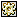Paste Behavior (Ctrl + V)

 Compatibilities: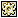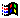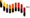This item has not yet been rated

Author: Macromedia (website)

From Technote #14130 at Macromedia.com

 property spriteNum, pWhichSprite, pWhichMember, pMemberType on beginSprite me   pWhichSprite = sprite(me.spriteNum)   pWhichMember = pWhichSprite.member   pMemberType = pWhichMember.type end on keyDown me   if (the commandDown) then     case (TRUE) of       (the commandDown AND the keyPressed = "v") : clipboardpaste me     end case   else     pass   end if end on clipboardPaste me   clipBoardRef = member "clipboard temp"   pasteClipBoardInto member "clipboard temp"   clipBoardRef.name = "clipboard temp"   charCount = member("Clipboard temp").char.count   if pMemberType = #text then     whichSelection = member(pWhichMember)Selection     num1 = whichSelection     num2 = whichSelection     if num1 <> num2 then       if num1 = 0 then         member(pWhichMember)Char[1..num2] = member("Clipboard temp").text         member(pWhichMember)Selection = [0, charCount]       else         member(pWhichMember)Char[(num1 + 1)..num2] = member("Clipboard temp")Text         member(pWhichMember)Selection = [num1, (num1 + charCount)]       end if     else       if num1 = 0 then         x = member(pWhichMember)Char         member(pWhichMember)Char = member("Clipboard temp")Text & x         member(pWhichMember)Selection = [0, charCount]       else         x = member(pWhichMember)Char[num1]         member(pWhichMember)Char[num1] = x & member("Clipboard temp")Text         member(pWhichMember)Selection = [num1, (num1 + charCount)]       end if     end if   end if   if pMemberType = #field then     num1 = the selStart     num2 = the selEnd     if num1 <> num2 then       if num1 = 0 then         member(pWhichMember)Char[1..num2] = member("Clipboard temp")Text         the selStart = 0         the selEnd = charCount       else         member(pWhichMember)Char[(num1 + 1)..num2] = member("Clipboard temp")Text         the selStart = num1         the selEnd = (num1 + charCount)       end if     else       if num1 = 0 then         x = member(pWhichMember)Char         member(pWhichMember)Char = member("Clipboard temp")Text & x         the selStart = 0         the selEnd = charCount       else         x = member(pWhichMember)Char[num1]         member(pWhichMember)Char[num1] = x & member("Clipboard temp")Text         the selStart = num1         the selEnd = (num1 + charCount)       end if     end if   end if end

 Contact MMI 36 South Court Sq Suite 300 Newnan, GA 30263 USA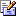Send e-mail